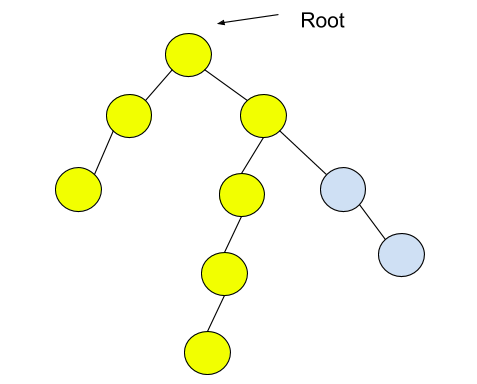New update is available. Click here to update.
Last Updated: 7 Dec, 2020
##### Diameter Of Binary Tree
Easy
Problem statement#### Return the length of the diameter of the tree.

##### Note :
``````The diameter of a binary tree is the length of the longest path between any two end nodes in a tree.

The number of edges between two nodes represents the length of the path between them.
``````
##### Example :
``````Input: Consider the given binary tree:
````````````Output: 6

Explanation:
Nodes in the diameter are highlighted. The length of the diameter, i.e., the path length, is 6.
``````

##### Input Format:
``````The only line contains elements in the level order form. The line consists of values of nodes separated by a single space. In case a node is null, we take -1 in its place.

For example, the input for the tree depicted in the below image will be:
````````````1
2 3
4 -1 5 6
-1 7 -1 -1 -1 -1
-1 -1

Explanation :
Level 1 :
The root node of the tree is 1

Level 2 :
Left child of 1 = 2
Right child of 1 = 3

Level 3 :
Left child of 2 = 4
Right child of 2 = null (-1)
Left child of 3 = 5
Right child of 3 = 6

Level 4 :
Left child of 4 = null (-1)
Right child of 4 = 7
Left child of 5 = null (-1)
Right child of 5 = null (-1)
Left child of 6 = null (-1)
Right child of 6 = null (-1)

Level 5 :
Left child of 7 = null (-1)
Right child of 7 = null (-1)

The first not-null node(of the previous level) is treated as the parent of the first two nodes of the current level. The second not-null node (of the previous level) is treated as the parent node for the next two nodes of the current level and so on.

The input ends when all nodes at the last level are null(-1).

The sequence will be put together in a single line separated by a single space. Hence, for the above-depicted tree, the input will be given as:

1 2 3 4 -1 5 6 -1 7 -1 -1 -1 -1 -1 -1
``````

##### Output Format:
``````Return a single integer i.e. the diameter of the tree.
``````

##### Note :
``````You do not need to print anything; it has already been taken care of. Just implement the given function.
``````Approaches

## 01ApproachThe basic idea of this approach is to break the problem into subproblems.

Now, there are three possible cases:

1. The diameter of the tree is present in the left subtree.
2. The diameter of the tree is present in the right subtree.
3. The diameter of the tree passes through the root node.

Let us define a recursive function, ‘getDiamter’, which takes the root of the binary tree as input parameter and returns the diameter of the given subtree rooted at the “root” node.

We can easily find the diameter of the left subtree and right subtree by recursion. The main task is to calculate the diameter of the tree corresponding to point 3 mentioned above.

From the above figure, we can observe that if the diameter passes through the root node, then it can be written as the length of the longest path between the leaves which passes through the root. And we can get that using the height of the left and right subtrees.

Now, assume a function, ‘getHeight,’ which returns the height of the subtree rooted at the “root” node.

The longest path length(i.e., Number of edges in the path) between the leaves can be written as:

1 + getHeight(left child of the root node) + getHeight(right child of the root node)

Algorithm

• If the ‘root’ node is NULL, return 0. The diameter of an empty tree is 0.
• Recur for the left subtree and store the diameter of the left subtree in a variable ‘leftDiameter’, i.e. ‘leftDiameter’ = getDiameter(left child of the root node)
• Similarly, recur for the right subtree and store the diameter of the right subtree in a variable ‘rightDiameter’ i.e. ‘rightDiameter’ = getDiameter(right child of the root node)
• Now, get the height of the left subtree and right subtree and store it in a variable.
• ‘leftHeight’ = getHeight(left child of the root node)
• ‘rightHeight’ = getHeight(right child of the root node)
• The diameter of the given tree will be the maximum of the following terms:
• ‘leftDiameter’
• ‘rightDiameter’
• 1 + ‘leftHeight’ + ‘rightHeight’
• Return the maximum of above terms i.e.max(leftDiameter, rightDiameter, 1 + leftHeight + rightHeight).